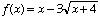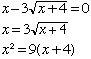I am having a problem with irrational algebraic functions. My name is Bucky and I am in the 9th grade taking Algebra II. Here is the multipart problem: Given f(x) = x-3* squareroot of x + 4 What does the f(x) intercept equal Find the two values for which f(x) = -5 Find the one value for which f(x) = -3 Can you please help show me the steps to arrive at the answers. This questions is from "Algebra and Trigonometry: Functions and Applications" by Paul A Foerster page 416. Hi Bucky, The three problems are very much the same. You have the functionand a number a and you are asked to solve f(x) = a. The three problems are a = 0, a = -3 and a = -5. The solution in each case starts with the same step. Isolate the term with the square root on one side of the equation, and then square both sides. Thus for the first problemNow simplify the expression and solve the quadratic x2 -9x -36 = 0 (x - 12)(x + 3) = 0 Thus x = 12 or x = -3 Finally check to see if both are answers to the original problem. (In this case only one is actually a solution.) Now try the second and third problems the same way. Cheers, Harley Go to Math Central